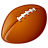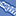# QlikView Documents

QlikView documentation and resources.

Announcements
Our next Qlik Insider session will cover new key capabilities. Join us August 11th REGISTER TODAY

# Set Analysis for Rolling PeriodsLuminary Alumni

## Set Analysis for Rolling Periods

Hi All,

Sometimes there may be requirements from users where they want to see the charts by rolling periods based on the selected periods, please check below for various scenarios.

Note: The format defined in SET DateFormat and your date field format in Qlikview Table should be same, then only this expressions works.

Last 5 Years Sales

Sum({<Year=, Quarter=, Month=, Week=, Date={‘>=\$(=YearStart(Max(Date), -4))<=\$(=Date(Max(Date)))’}>} Sales )

Last 6 Quarters Sales

Sum({<Year=, Quarter=, Month=, Week=, Date={‘>=\$(=QuarterStart(Max(Date), -5))<=\$(=Date(Max(Date)))’}>} Sales )

Last 12 Months Sales

Sum({<Year=, Quarter=, Month=, Week=, Date={‘>=\$(=MonthStart(Max(Date), -11))<=\$(=Date(Max(Date)))’}>} Sales )

Last 15 Weeks Sales

Sum({<Year=, Quarter=, Month=, Week=, Date={‘>=\$(=WeekStart(Max(Date), -14))<=\$(=Date(Max(Date)))’}>} Sales )

Last 10 Days Sales

Sum({<Year=, Quarter=, Month=, Week=, Date={‘>=\$(=Date(Max(Date)-9))<=\$(=Date(Max(Date)))’}>} Sales )

Note:

YTD - Year to Date

QTD - Quarter to Date

MTD - Month to Date

WTD - Week to Date

Last YTD for the period selected

Sum({<Year=, Quarter=, Month=, Week=, Date={‘>=\$(=YearStart(Max(Date), -1))<=\$(=AddYears(Max(Date), -1))’}>} Sales)

Last QTD for the period selected

Sum({<Year=, Quarter=, Month=, Week=, Date={‘>=\$(=QuarterStart(Max(Date), -1))<=\$(=AddMonths(Max(Date), -3))’}>} Sales)

Last MTD for the period selected

Sum({<Year=, Quarter=, Month=, Week=, Date={‘>=\$(=MonthStart(Max(Date), -1))<=\$(=AddMonths(Max(Date), -1))’}>} Sales)

Last WTD for the period selected

Sum({<Year=, Quarter=, Month=, Week=, Date={‘>=\$(=WeekStart(Max(Date), -1))<=\$(=Date(Max(Date) -7))’}>} Sales)

Hope this helps.

Regards,

Jagan.

Labels (6)

• ### QlikView ApplicationsLuminary Alumni

Thanks for sharing Jagan, these expression are in great demand and having them here will be very useful to lots of people I'm sure.

Sometimes you're required to do slightly more complex 'As Of' calculations. Last business day for example or perhaps you want a comparative MTD (if Today is the 15th of Sep you want to see results from the 1st to the 15th of August). If this is the case then you may want to check out my post

Calendar with flags making set analysis so very simple

Richard

QlikCentral.ComMVP

Jagan, Nice ArticleSpecialist IIContributor II

This is a really cool little set of set analysis. I (unfortunately) couldn't get it to work and I assume it's because my DateFormat setting is different than the field value's format for some reason.

I figured out a different way to do this. I simply set a variable for X time period in the past and then do the set analysis to evaluate the time period greater than that value.

So for instance, if I want to look back at the past 5 years, I set a variable for 5 years ago like so:

Let v5YearsAgo = Year(Yearstart( Today( ) ) )-4;

Then when I want to use this in set analysis, I simply evaluate it normally:

Sum({<Year={'>=\$(v5YearsAgo)'}>} GrossPay )

Hope that helps other folks that may have had trouble getting this to work.Creator

Thanks

for SharingContributor II

Really helpful, I will use this for my app.

ThanksContributor

Hi Jagan

Firstly, thanks for the beautiful post. However, I am not able to fully comprehend the details of it as i am just a beginner here.

I have two columns of information with me. One is the Incident ID (a field) and second one is  a Date Stamp [4/23/2018 6:02:11 AM]  on which the Incident was Created. Like this i have data starting from 1-1-2018 onward until today.

My questions is, how can I calculate and display the Last 12 month COUNT in bar chart. How can i just display ONLY the last 12 month on my X-Axis and the respective count of incident for those months.

Formula for x-Axis

Formula for Count:

for the count i used the below formula but nothing come up in the graph

Count({< Month=[Submit Date]{>=\$(=MonthStart(Max([Submit Date]), -11))<=\$(=Date(Max([Submit Date])))}>} [Incident ID])

Your help will be much appreciated.

Cheer - NitinContributor III

Hi Nitin,

Better late than never.

Here is what I would write:

``Count({\$<[Submit Date]={">\$(=MonthStart(AddMonths(Max([Submit Date]), -12)))<=\$(=MonthStart(Max([Submit Date])))"}>}[Incident ID])``Contributor III

Hi all,

I have a question basing what you've been written above.  I would like to count only data for last month from todays date, only for status "Open" I'm just qlik begginner and will appreciate your support

 Doc No Date Last Action Status 10312311 10/30/2019 Open 12315432 1/1/2019 Validated 23432312 10/21/2019 Open 42355312 10/2/2019 Validated 18712329 9/20/2019 Open 12452004 10/8/2019 Processed 12314322 9/30/2019 OpenContributor III

Hi Maciej29,

Can't you use the global filters? You could select Status=Open and Date Last Action corresponding to the wished month.

If you want to use Set Analysis, I would write it like this:

``Count(distinct {\$<Status={"Open"}, DateLastAction={">=\$(=MonthStart(Today()))<\$(=MonthStart(AddMonths(Today(), 1)))"}>}[Doc No])``
Version history
Revision #:
1 of 1
Last update:
‎2015-09-02 11:32 PM
Updated by: